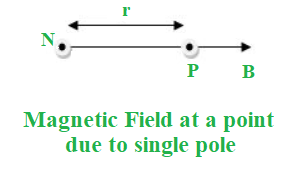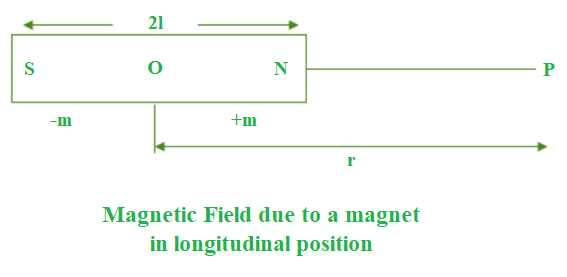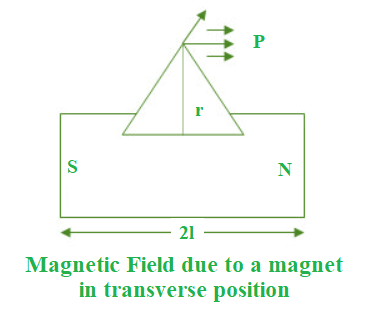# Magnetization and Magnetic Intensity

• Last Updated : 21 Sep, 2021

We’ve all had fun with magnets as kids. Some of us are now even playing with them! What makes them magnetic though? Why aren’t there magnetic fields in all materials and substances? Have you ever given it any thought? The subjects of magnetization and magnetic intensity will be covered in this chapter.

### Magnetization

Magnetization is caused by a magnetic moment, as we all know. This is caused by the mobility of electrons in atoms. The response of a substance to an external magnetic field determines its net magnetization.

Attention reader! All those who say programming isn't for kids, just haven't met the right mentors yet. Join the  Demo Class for First Step to Coding Course, specifically designed for students of class 8 to 12.

The students will get to learn more about the world of programming in these free classes which will definitely help them in making a wise career choice in the future.

It also takes into account any imbalanced magnetic dipole moment that the material may have due to the mobility of its electrons, as previously indicated. The idea of magnetization aids in the classification of materials according to their magnetic properties.

We’ll learn more about magnetization and the idea of magnetic intensity in this part. The arrangement of the atoms inside a material determines a magnet’s magnetic behaviour. This is what we’ll be discussing in this article. The magnetization (M) of the material is equal to the net magnetic moment per unit volume of that material.

In terms of mathematics,

M = mnet ⁄ V

Let’s take a look at the case of a solenoid. If we consider a solenoid with n turns per unit length and a current I flowing through it, the magnetic field in the solenoid’s interior may be expressed as,

B0 = μ0 n I

where, µ0 is the constant permeability of a vacuum.

If we fill the solenoid’s inside with a non-zero magnetization material, the field within the solenoid must be higher than before. Inside the solenoid, the net magnetic field B may be written as,

B = B0 + Bm

where, Bm is the field provided by the core material.

It is proportional to the material’s magnetization in this case. In terms of mathematics,

Bm = μ0 M

Let us now look at another concept: a material’s magnetic intensity. A material’s magnetic intensity can be expressed as,

H = (B ⁄ μ0) − M

The total magnetic field may alternatively be defined as, as shown by this equation.

B = μ0 (H + M)

H denotes the magnetic field owing to external variables such as the current in the solenoid, whereas M denotes the magnetic field due to the nature of the core. The latter amount, M, is influenced by external factors and is given by.

M = χ H

where, χ is the material’s magnetic susceptibility.

Magnetic susceptibility is a measurement of a material’s reaction to an external field. For paramagnetic materials, the magnetic susceptibility is small and positive, while for diamagnetic materials, it is tiny and negative.

Substitute the value of M in the equation of B.

B = μ0 (H + χ H) = μ0 (1 + χ) H

= μ0 μr H = μ H

Here, μr is the relative magnetic permeability of the material which is comparable to the dielectric constants in electrostatics. The magnetic permeability is defined as follows:

μ = μ0 μr = μ0 (1 + χ)

### Magnetic Intensity

The arrangement of the atoms inside a material determines a magnet’s magnetic behaviour. When a ferromagnetic substance is subjected to a strong external magnetic field, it experiences a torque, which causes the substance to align itself in the direction of the applied magnetic field and therefore become strongly magnetised in that direction.

The force that a unit north – pole experiences when it is put in a magnetic field is described as the magnetic intensity at that location. The magnetic field strength at P owing to a single pole is given by:The magnetic field B, we argue, may be expressed as:

B = (μ0 ⁄ 4 π) (m / π r2)

We know, μ0 ⁄ 4 π = 10−7

⇒ B = 10−7 × (m / π r2)

where, m is the pole strength.

### The Intensity of Magnetic Field due to a Magnet at Different Points

• In Longitudinal PositionThe +⁄-m is the magnitude of the south and north poles, respectively. r is the distance between point P and the magnet’s centre. The length of the bar magnet is denoted by the letter l. The magnetic field intensity at point P is given by:

B = (μ0 ⁄ 4π) (2M r ⁄ r2 − l2)

where, M is the magnetic moment and (2ml) is the length of the magnetic moment.

For a small magnet, r2 >> l2:

B = (μ0 ⁄ 4π) (2M ⁄ r3)

• In Transverse PositionThe magnetic field intensity at point P is given by,

B = (μ0 ⁄ 4π) (M ⁄(r2 + l2)3 ⁄ 2)

For a small magnet, r2 >> l2:

B = (μ0 ⁄ 4π) (M ⁄ r3)

⇒ B ∝ 1 ⁄ r3

### Definition of Intensity of Magnetisation

When a magnet is put in a magnetic field, it changes its magnetic moment. The Intensity of Magnetisation is defined as the change in the magnetic moment per unit volume.

The formula of Intensity of Magnetisation is given as:

I = M ⁄ V

Magnetic moment, M = m x

Volume, V= A x

⇒ I = m x ⁄ A x = m ⁄ A

where,

• m is the pole strength,
• A is the area of cross-section,
• x is the length of the magnet, &
• I is the intensity of magnetisation.

### Sample Questions

Question 1: What is the distinction between Magnetic Intensity and Magnetisation Intensity?

The magnetic intensity of a magnet specifies the forces experienced by its poles in a magnetic field, whereas the intensity of magnetization explains the change in a magnet’s magnetic moment per unit volume.

Question 2: What exactly do you mean when you say “induced magnetization”?

Induced magnetization is a method of making a non-magnetic substance magnetic. When you put it under the influence of an external magnetic field, you may do so.

Question 3: What is the formula for magnetic intensity, and what does it mean?

The magnetic field strength at a particular place can be expressed as a vector quantity known as the magnetic intensity, abbreviated as H. Letter H, on the other hand, equals nI. The magnetic intensity unit is given as A ⁄ m, and its dimensions are [L−1M0T0I1].

Question 4: What is the definition of magnetic intensity?